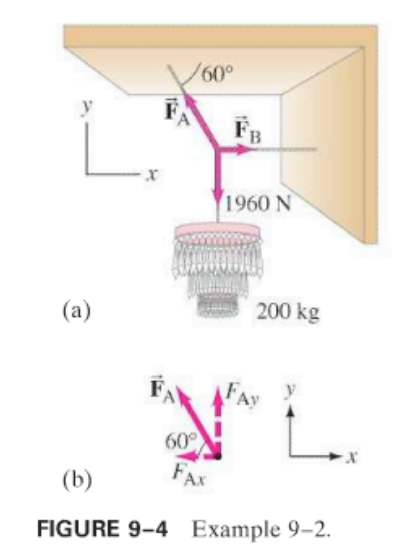×
Get Full Access to Physics: Principles With Applications - 6 Edition - Chapter 9 - Problem 5p
Get Full Access to Physics: Principles With Applications - 6 Edition - Chapter 9 - Problem 5p

×

# Two cords support a chandelier in the manner shown in Fig.ISBN: 9780130606204 3

## Solution for problem 5P Chapter 9

Physics: Principles with Applications | 6th Edition

• Textbook Solutions
• 2901 Step-by-step solutions solved by professors and subject experts
• Get 24/7 help from StudySoup virtual teaching assistantsPhysics: Principles with Applications | 6th Edition

4 5 1 305 Reviews
25
4
Problem 5P

Two cords support a chandelier in the manner shown in Fig. 9–4 except that the upper wire makes an angle of 45° with the ceiling. If the cords can sustain a force of 1550 N without breaking, what is the maximum chandelier weight that can be supported?Step-by-Step Solution:

SolutionStep 1 of 3 The impulse is large force acts on a body in short interval of timeWe know that pF t pF =k t where kis proportional constant its value is 1F = mv because linear momentum p=mv t mvF = t here m is constantF t=mvF t=p where p=p -p f iJ = p fp i So The impulse is defined as the change in the momentum.The expression for the impulse is expressed as,J = p -p f iHere,p ii the initial momentum, and p is thf final momentum.

Step 2 of 3

Step 3 of 3

##### ISBN: 9780130606204

Unlock Textbook Solution